# RS Aggarwal Solutions for Class 9 Chapter 1: Number Systems Exercise 1D

## RS Aggarwal Solutions for Class 9 Maths Exercise 1D PDF

Exam preparations can be made simpler for the students by providing them with exercise-wise solutions in PDF format. Subject experts with high conceptual knowledge prepare the solutions for each exercise to make it easier for the students to understand it. Exercise 1D in the RS Aggarwal Solutions for Class 9 Maths has problems based on real numbers and their properties like addition and multiplication. This exercise mainly contains many number of problems in order to help the students to solve it faster with good time management. RS Aggarwal Solutions for Class 9 Maths Chapter 1 Number Systems Exercise 1D are provided here.

## RS Aggarwal Solutions for Class 9 Chapter 1: Number Systems Exercise 1D Download PDF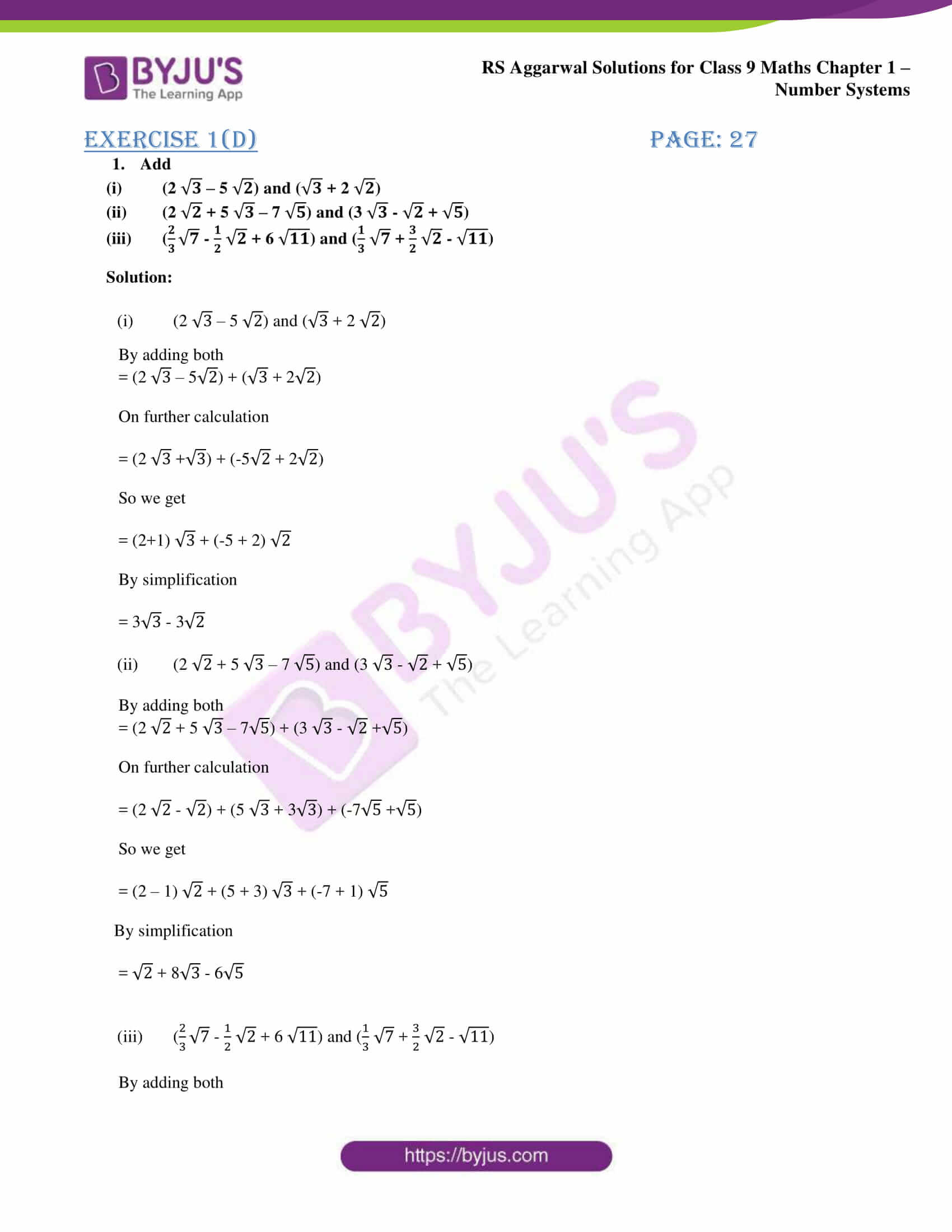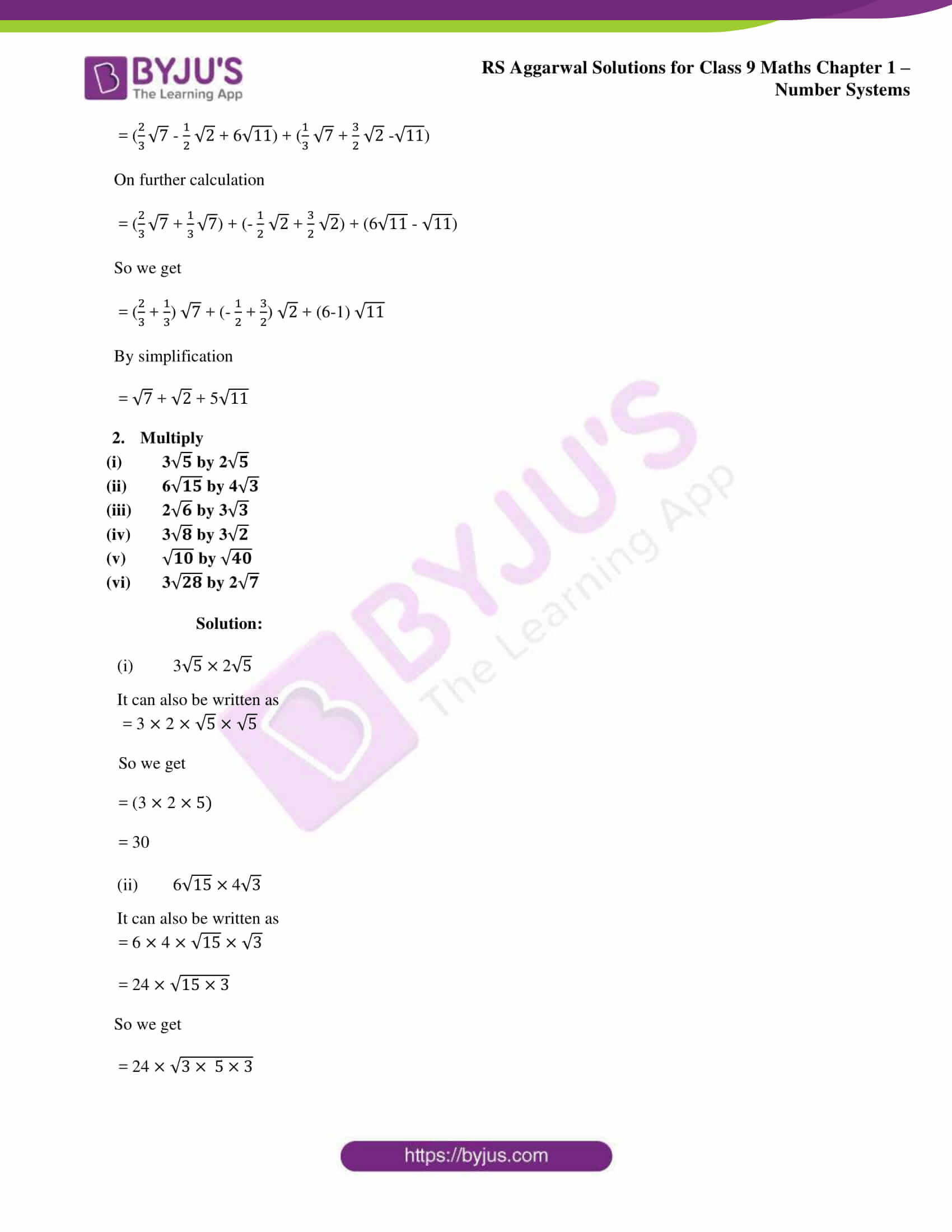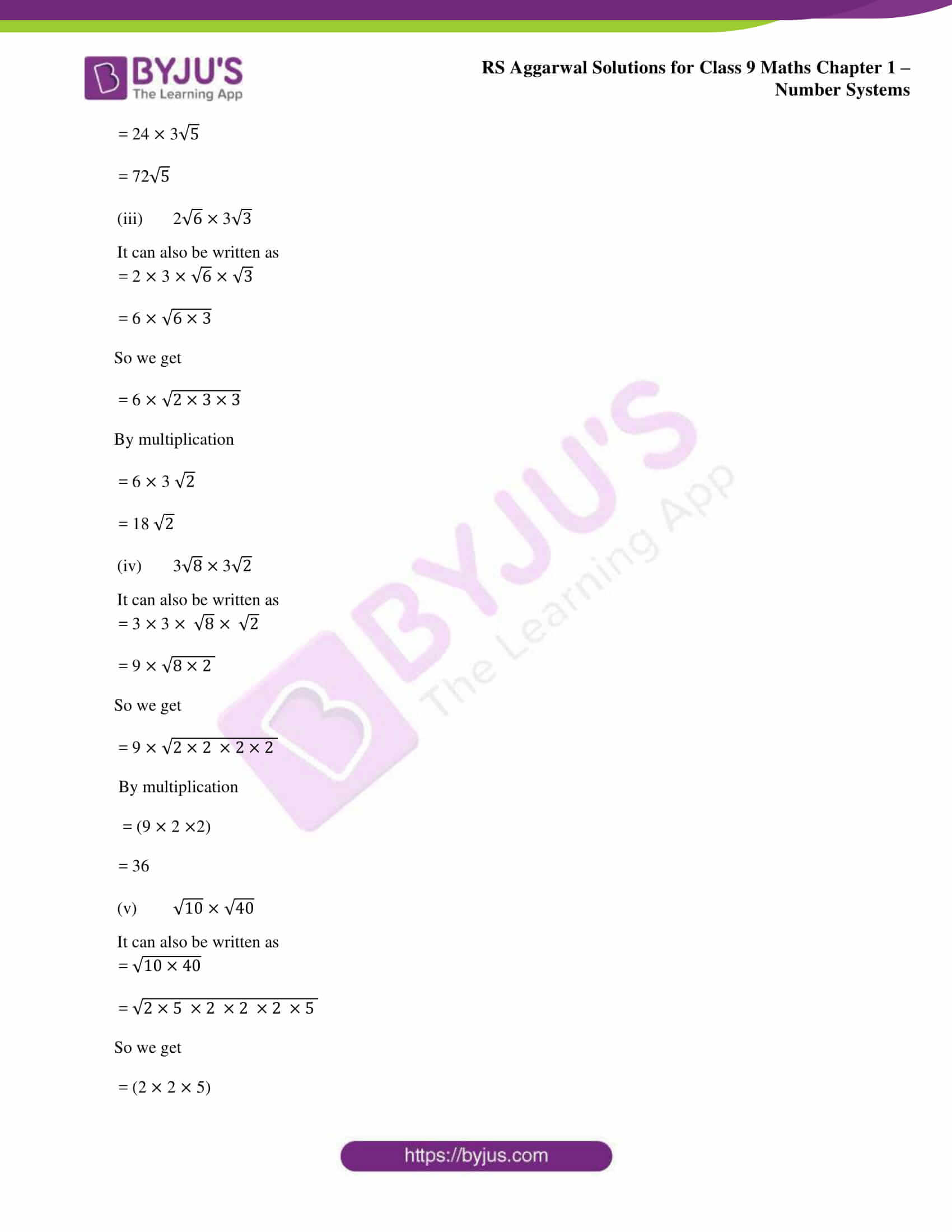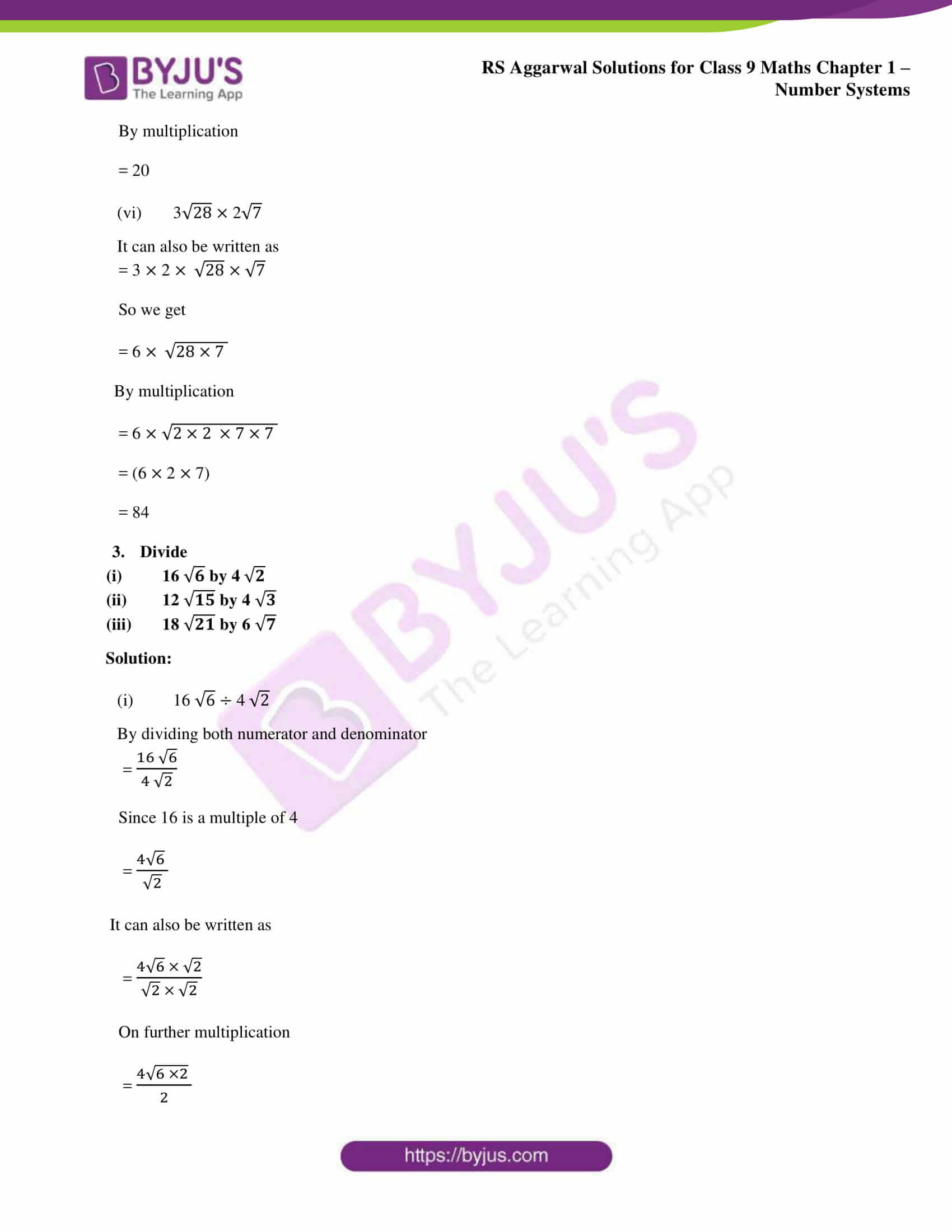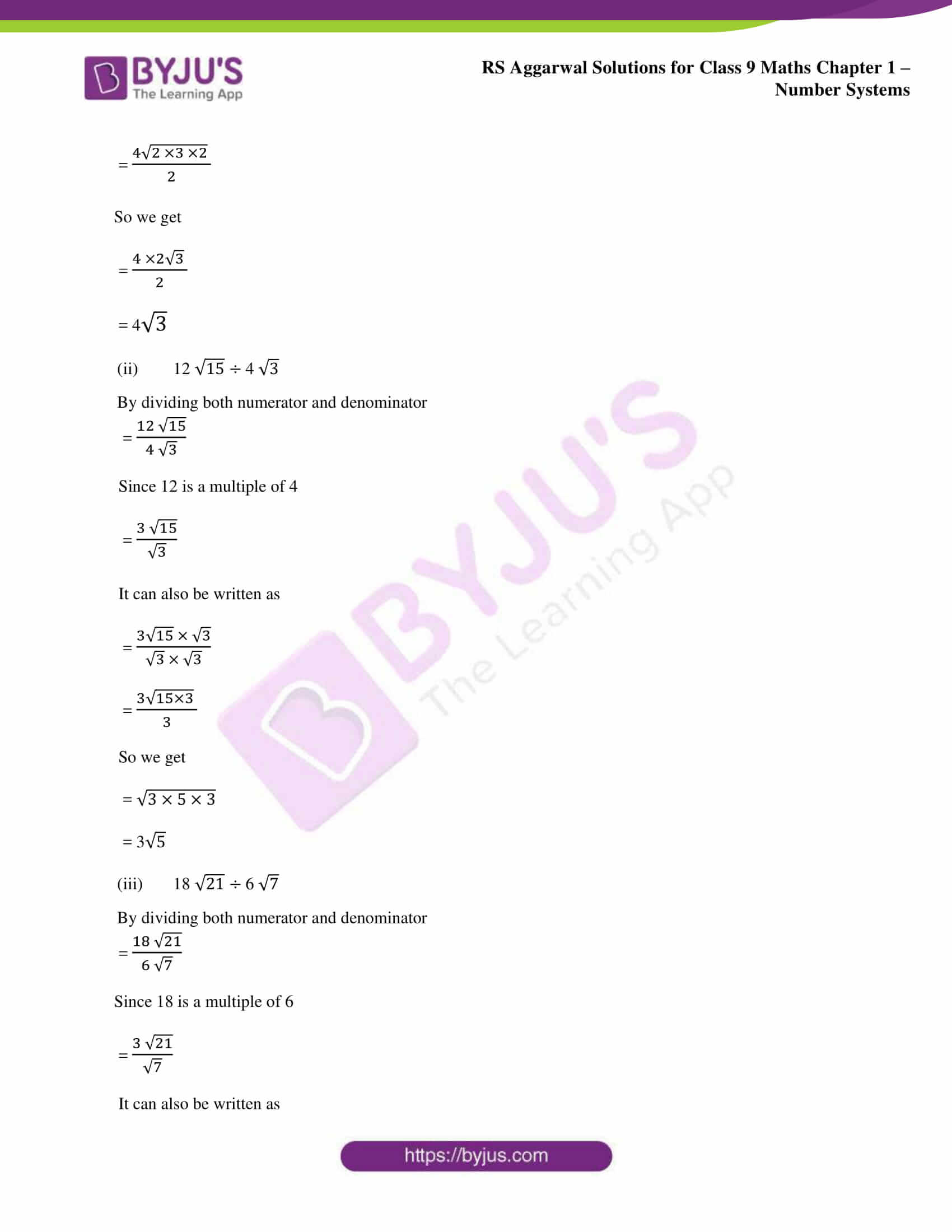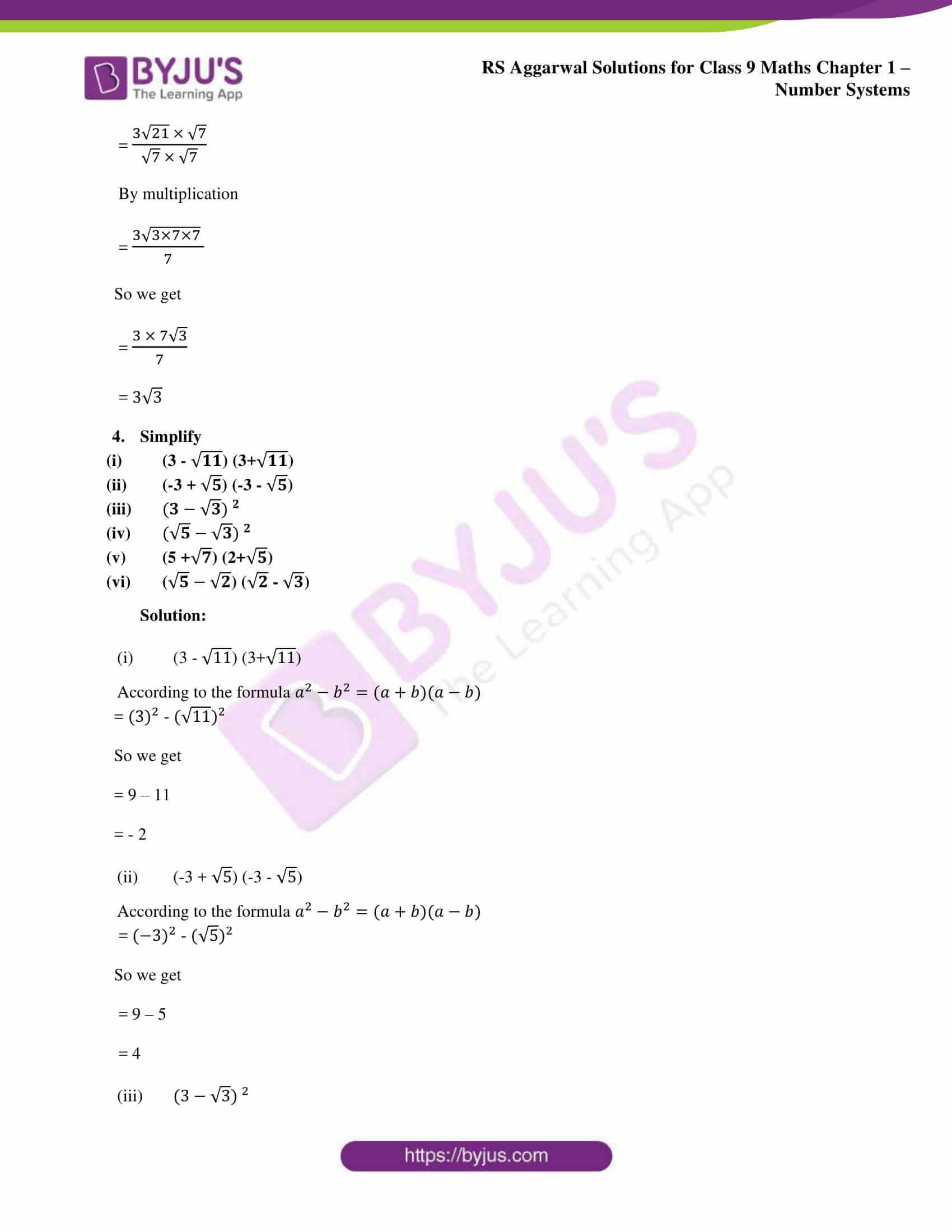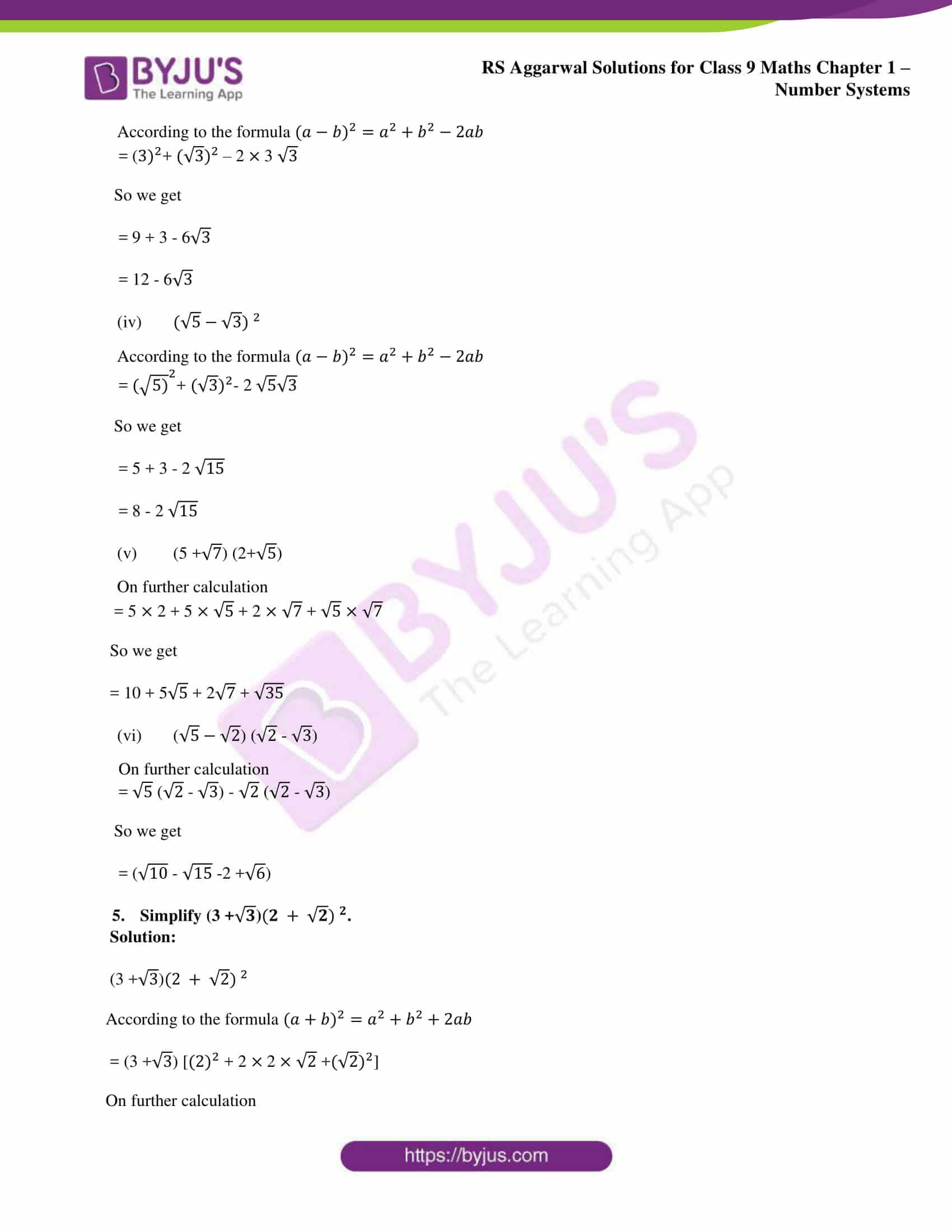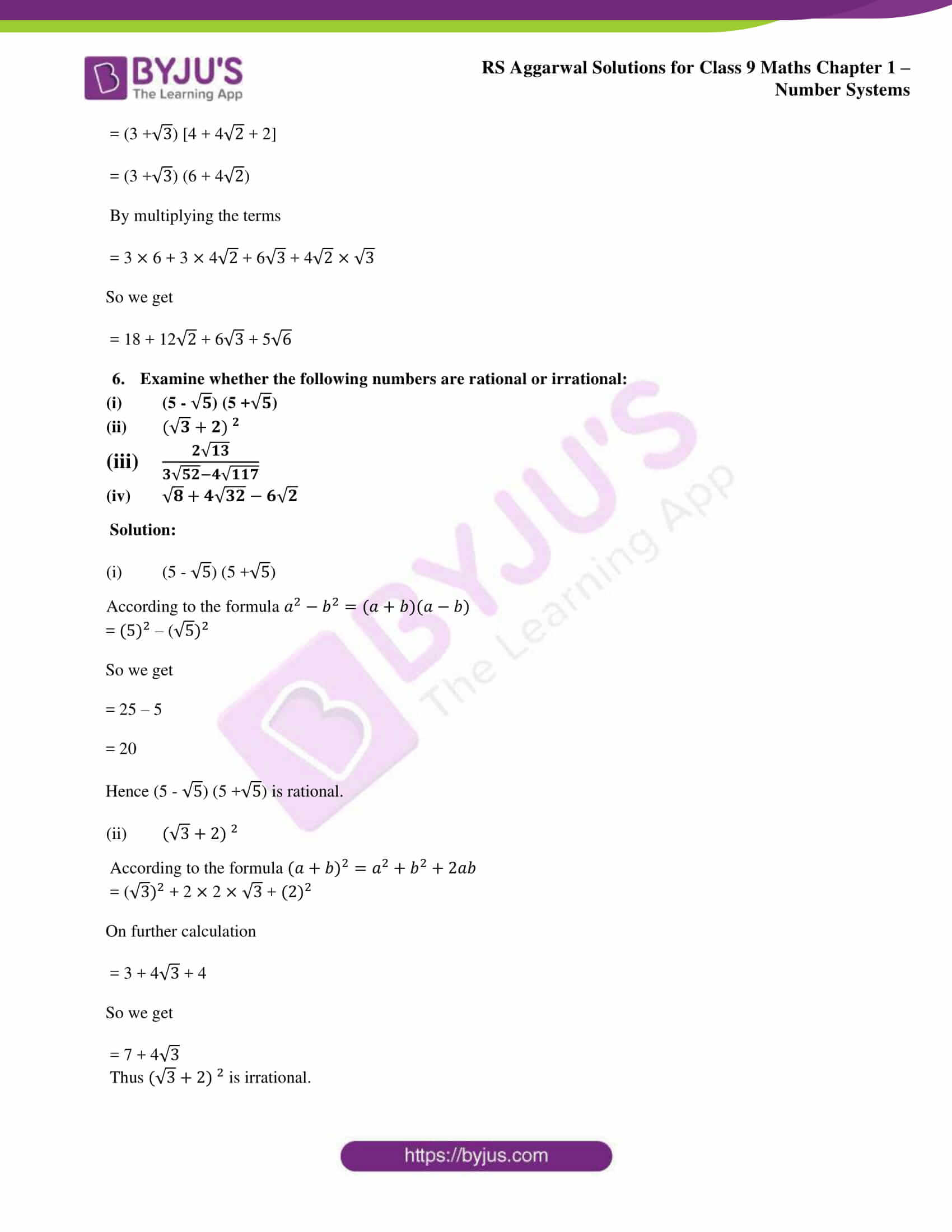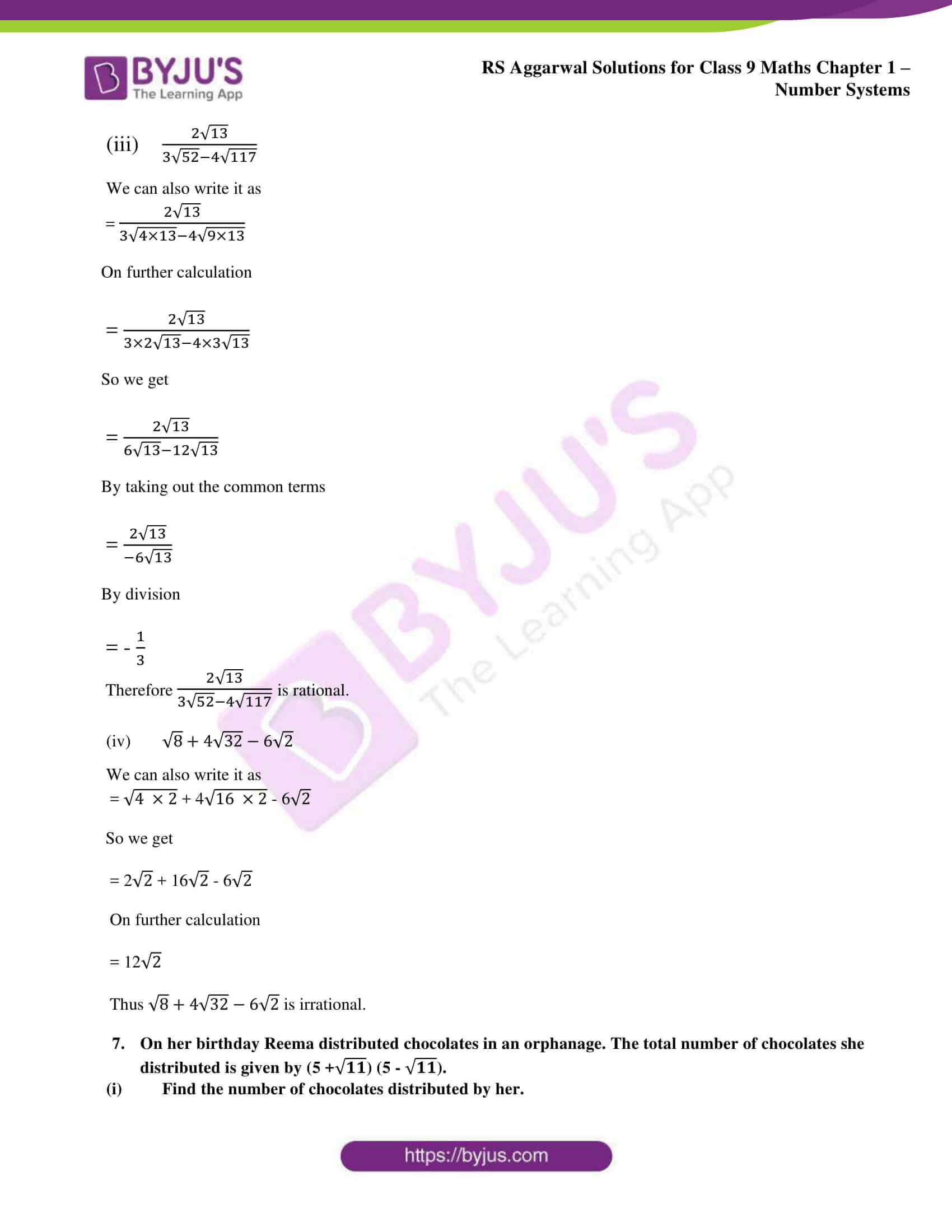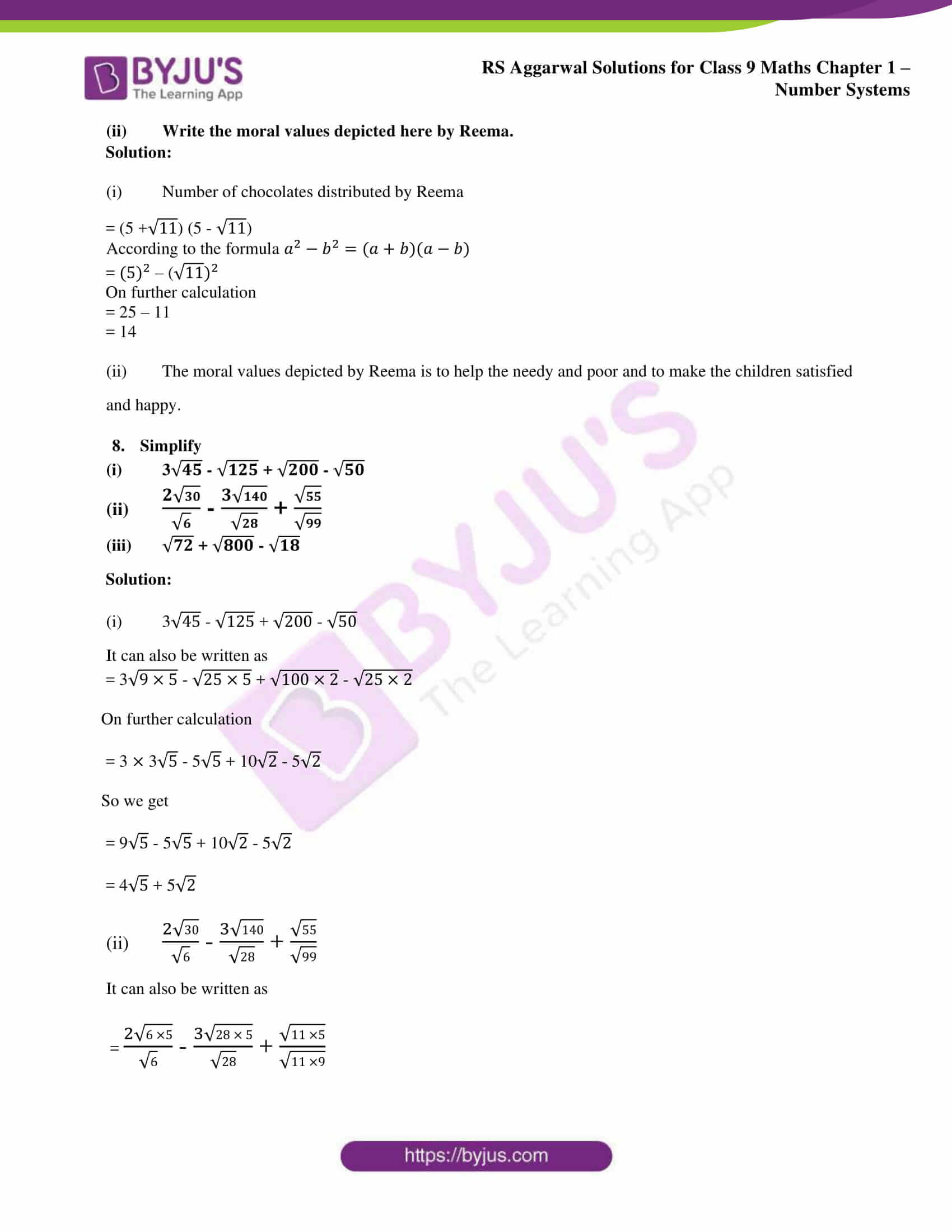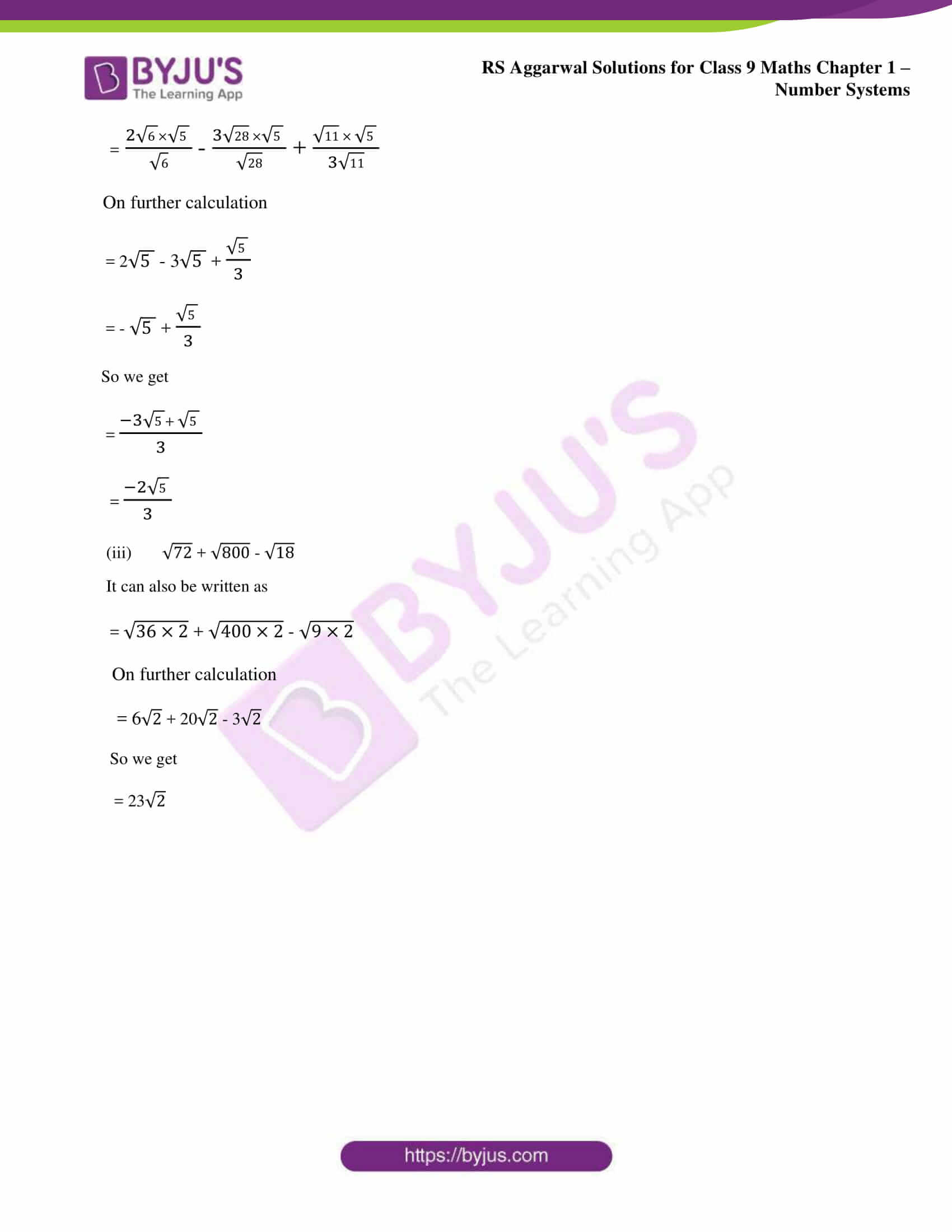### Access other exercise solutions of class 9 Maths Chapter 1: Number Systems

Exercise 1A Solutions 9 Questions

Exercise 1B Solutions 5 Questions

Exercise 1C Solutions 14 Questions

Exercise 1E Solutions 10 Questions

Exercise 1F Solutions 25 Questions

Exercise 1G Solutions 18 Questions

### RS Aggarwal Solutions Class 9 Maths Chapter 1 – Number Systems Exercise 1D

RS Aggarwal Solutions Class 9 Maths Chapter 1 Number Systems Exercise 1D has problems with answers covering some of the major topics like real numbers and their properties like multiplication and addition.

### Key features of RS Aggarwal Solutions for Class 9 Maths Chapter 1: Number Systems Exercise 1D

• The exercise solutions are solved by the experts using different methods and techniques along with examples according to the RS Aggarwal textbook.
• Exercise wise solutions are available in PDF format to make it easy for the exam preparation of the students.
• Regular practice of solutions during revision can develop problem solving skills of students to face the exam.
• Practicing many number of questions in each exercise will help students understand the steps to be followed while solving it.